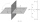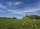On line

On line p: x = 4 + t, y = 3 + 2t, t is R, find point C, which has the same distance from points A [1,2] and B [-1,0].

Result

x =  2
y =  -2

Solution:Leave us a comment of example and its solution (i.e. if it is still somewhat unclear...):Be the first to comment!To solve this example are needed these knowledge from mathematics:

For Basic calculations in analytic geometry is helpful line slope calculator. From coordinates of two points in the plane it calculate slope, normal and parametric line equation(s), slope, directional angle, direction vector, the length of segment, intersections the coordinate axes etc. Looking for help with calculating roots of a quadratic equation? Do you have a linear equation or system of equations and looking for its solution? Or do you have quadratic equation? Pythagorean theorem is the base for the right triangle calculator.

Next similar examples:

1. Parametric equationFind the parametric equation of a line with y-intercept (0,-4) and a slope of -2.
2. Symmetry by planeDetermine the coordinates of a image of point A (3, -4, -6) at a symmetry that is determined by the plane x-y-4z-13 = 0
3. Sphere equationObtain the equation of sphere its centre on the line 3x+2z=0=4x-5y and passes through the points (0,-2,-4) and (2,-1,1).
4. Distance problem 2A=(x,2x) B=(2x,1) Distance AB=√2, find value of x
5. Circle - AGFind the coordinates of circle and its diameter if its equation is: ?
6. Evaluation of expressionsIf a2-3a+1=0, find (i)a2+1/a2 (ii) a3+1/a3Find the roots of the quadratic equation: 3x2-4x + (-4) = 0.
8. Right angled triangleHypotenuse of a right triangle is 17 cm long. When we decrease length of legs by 3 cm then decrease its hypotenuse by 4 cm. Determine the size of legs.
9. Theorem proveWe want to prove the sentence: If the natural number n is divisible by six, then n is divisible by three. From what assumption we started?
10. Square root 2If the square root of 3m2 +22 and -x = 0, and x=7, what is m?
11. CathetiThe hypotenuse of a right triangle is 41 and the sum of legs is 49. Calculate the length of its legs.
12. RTriangle 17The hypotenuse of a right triangle is 17 cm. If you decrease both two legs by 3 cm you will reduce the hypotenuse by 4 cm. Determine the length of this legs.
13. RT and circlesSolve right triangle if the radius of inscribed circle is r=9 and radius of circumscribed circle is R=23.
14. RootsDetermine the quadratic equation absolute coefficient q, that the equation has a real double root and the root x calculate: ?
15. DiscriminantDetermine the discriminant of the equation: ?
16. EquationEquation ? has one root x1 = 8. Determine the coefficient b and the second root x2.
17. Solve 3Solve quadratic equation: (6n+1) (4n-1) = 3n2